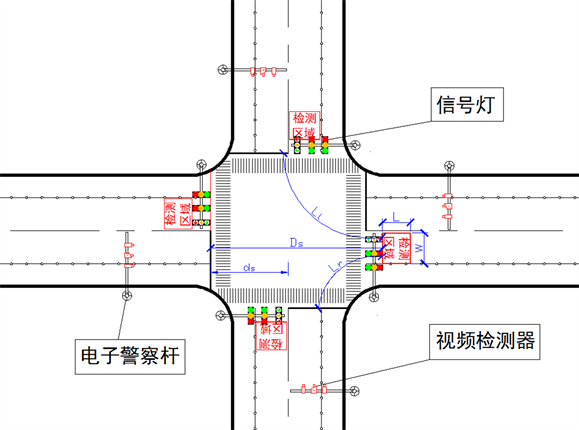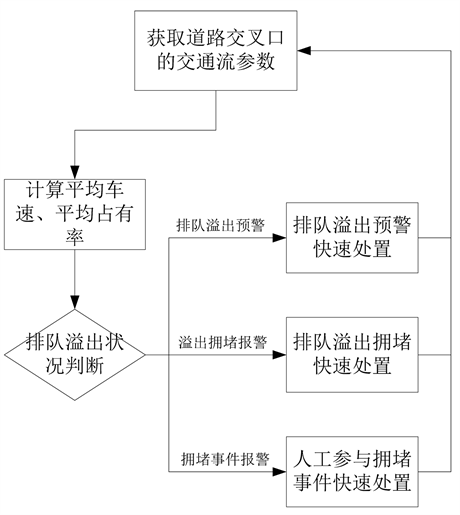# 基于智能检测的单点控制交叉口出口交通流溢出三级预警The Three Level Warning of Queue Overflow of Single Point Control Intersection Based on Intelligent Detection

• 全文下载: PDF(513KB)    PP.288-293   DOI: 10.12677/OJTT.2018.74035
• 下载量: 231  浏览量: 300

In this paper, in view of the traffic congestion caused by the traffic flow queuing overflow, which is easy to occur at the peak period of traffic flow, this paper proposes a scheme to set up the detection area in the exit channel. The traffic flow queuing overflow is divided into three cases through the parameter judgment, which is obtained in real-time traffic flow. The three cases include queuing overflow warning and queuing overflow congestion alarm and congestion event alarm. The detection scheme proposed in this paper overcomes the disadvantages of low data accuracy in the existing technology, which is caused by the impact of the vehicles in and out of the road opening. Classifying the queuing overflow events is more consistent with the complexity of the actual traffic operation.

1. 引言

2. 出口交通流溢出智能检测系统设计

2.1. 确定检测区域

2.2. 进行检测器选型

2.3. 确定安装位置及安装方式

2.4. 检测器与信号机通信要求

2.5. 获取交通流参数

3. 出口交通流溢出三种情况分析Figure 1. The sketch of fixing video detector and mapping detection area

4. 出口交通流溢出三级报警检测

4.1. 三级报警检测流程

4.2. 计算平均车速和平均占有率

1) 计算当前周期内车辆通过检测区域的平均时间占有率，通过平均时间占有率才可以计算出平均行驶速度，计算公式如下：

${t}_{occ}^{n}={t}_{off}^{n}-{t}_{in}^{n}$

$\stackrel{¯}{{t}_{occ}}=\frac{\underset{n=1}{\overset{N}{\sum }}{t}_{occ}^{n}}{T}$Figure 2. The flow chart of technical scheme

2) 计算当前周期内车辆通过检测区域的平均行驶速度，计算公式如下：

${v}_{n}=\frac{L}{{t}_{occ}^{n}}$

$\stackrel{¯}{v}=\frac{\underset{n=1}{\overset{N}{\sum }}{v}_{n}}{N}$

3) 计算某相位绿灯时段内越过停止线的车辆能够全部驶入出口引道的临界通行车速，计算公式如下：

${V}_{通}^{pha}=\frac{\underset{dir}{\sum }\left(\frac{{Q}_{in}^{dir}}{a}×\left({l}_{car}+{s}_{0}\right)+{D}^{dir}\right)×\frac{{Q}_{in}^{dir}}{\underset{dir}{\sum }{Q}_{in}^{dir}}}{{G}^{pha}+{Y}^{pha}}$

4) 计算车辆通过检测区域而不引起发生排队溢出状况的临界通行时间占有率，计算公式如下：

${t}_{通}=\frac{L}{{V}_{通}^{pha}}$

${T}_{通}=\frac{{t}_{通}×N}{T}$

5) 计算某相位绿灯时段内发生排队溢出拥堵时的临界拥堵行驶速度，计算公式如下：

${V}_{堵}^{pha}=\frac{\underset{dir}{\sum }\left(\frac{{Q}_{in}^{dir}}{a}×\left({l}_{car}+{s}_{0}\right)+{D}_{中}^{dir}\right)×\frac{{Q}_{in}^{dir}}{\underset{dir}{\sum }{Q}_{in}^{dir}}}{{G}^{pha}+{Y}^{pha}}$

6) 计算某相位绿灯时段内发生排队溢出拥堵的临界拥堵时间占有率，计算公式如下：

${t}_{堵}=\frac{L}{{V}_{堵}^{pha}}$

${T}_{堵}=\frac{{t}_{堵}×N}{T}$

4.3. 排队溢出状况判断

1) 由第2节排队溢出预警定义推导出排队溢出状况发生条件：当路口某一个方向发生 ${V}_{堵}^{pha}<\stackrel{¯}{v}<{V}_{通}^{pha}$ 并且 ${T}_{通}<\stackrel{¯}{{t}_{occ}}<{T}_{堵}$ 时，发生排队溢出预警。

2) 由第2节排队溢出拥堵定义推导出拥堵状况发生条件：当路口某一个方向发生 $0<\stackrel{¯}{v}\le {V}_{堵}^{pha}$ 并且 ${T}_{堵}\le \stackrel{¯}{{t}_{occ}}$ 时，发生溢出拥堵报警。

3) 由第2节路口拥堵事件报警定义推导出拥堵事件报警发生条件：当路口溢出拥堵方向发生 $\stackrel{¯}{v}=0$ 并且 $\stackrel{¯}{{t}_{occ}}>C$ (C为信号控制周期时长)时，进行路口拥堵事件报警。

5. 结语

  李萌萌. 预防交叉口排队溢出的交通信号控制方法研究[D]: [硕士学位论文]. 哈尔滨: 哈尔滨工业大学, 2015: 1-2.  张博, 宋志洪, 梁子君, 于海洋, 宜林川. 一种用于道路交叉口车辆排队溢出状态的交通信号优化控制方法[P]. 中国: CN104157152A, 2014-11-19.  林赐云, 龚勃文, 曲鑫. 一种过饱和交通流交叉口排队溢出防控方法[P]. 中国: CN104966402A, 2015-10-07.  高万宝, 吴先会, 李慧玲. 一种基于视频的单点自优化信号控制方法及装置[P]. 中国: CN105118310A, 2015-12-02.